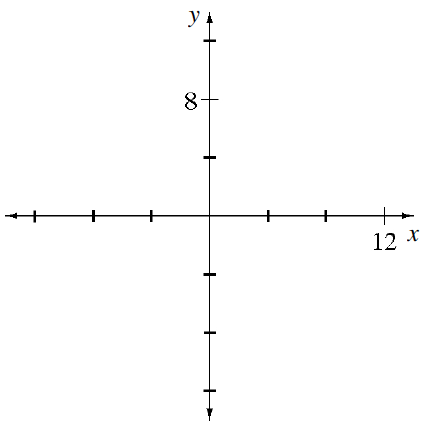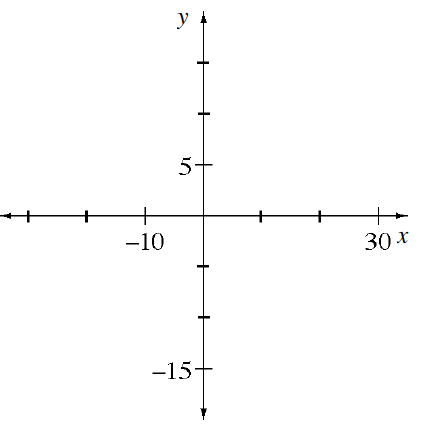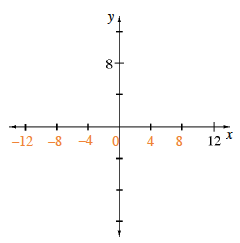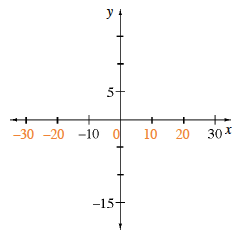### Home > MC1 > Chapter 6 > Lesson 6.2.5 > Problem6-98

6-98.

Copy the axes below and complete the scales.

1.1.Remember that where the $x\text{-}$ and $y\text{-axes}$ intersect is always $0$. To scale the $x\text{-axis}$ in part (a), you should try dividing $12$ by $3$, since that is the number of spaces between $0$ and $12$.

The $x\text{-axes}$ for parts (a) and (b) are labeled below. Were you able to scale the $y\text{-axes}$?